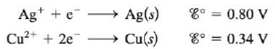# A galvanic cell is based on the following half-reactions: In this cell, the silver compartment contains a silver electrode and excess AgCl( s ) (K sp = 1.6 × 10 −10 ), and the copper compartment contains a copper electrode and [Cu 2+ ] = 2.0 M . a. Calculate the potential for this cell at 25°C. b. Assuming 1.0 L of 2.0 M Cu 2+ in the copper compartment, calculate the moles of NH 3 that would have to be added to give a cell potential of 0.52 Vat 25°C (assume no volume change on addition of NH 3 ). Cu 2 + ( a q ) + 4 NH 3 ( a q ) ⇌ Cu ( NH 3 ) 4 2 + ( a q ) K = 1.0 × 10 13### Chemistry: An Atoms First Approach

2nd Edition
Steven S. Zumdahl + 1 other
Publisher: Cengage Learning
ISBN: 9781305079243

#### Solutions

Chapter
Section### Chemistry: An Atoms First Approach

2nd Edition
Steven S. Zumdahl + 1 other
Publisher: Cengage Learning
ISBN: 9781305079243
Chapter 17, Problem 149CP
Textbook Problem
1 views

## A galvanic cell is based on the following half-reactions:In this cell, the silver compartment contains a silver electrode and excess AgCl(s) (Ksp = 1.6 × 10−10), and the copper compartment contains a copper electrode and [Cu2+] = 2.0 M.a. Calculate the potential for this cell at 25°C.b. Assuming 1.0 L of 2.0 M Cu2+ in the copper compartment, calculate the moles of NH3 that would have to be added to give a cell potential of 0.52 Vat 25°C (assume no volume change on addition of NH3). Cu 2 + ( a q ) + 4 NH 3 ( a q ) ⇌ Cu ( NH 3 ) 4 2 + ( a q ) K = 1.0 × 10 13

(a)

Interpretation Introduction

Interpretation: The cell potential for the given galvanic cell and the number of moles of NH3 that would have to be added to give a given cell potential at 2οC are to be calculated.

Concept introduction: The limited form of galvanic cell is concentration cell consists of two equivalent half-cells of the same composition. Cell potential is defined as the measure of energy per unit charge available from the redox reaction to carry out the reaction. By measuring cell potential the voltage that exists between the two half cells is calculated.

To determine: The cell potential for the given galvanic cell at 2οC .

### Explanation of Solution

Explanation

The cell potential for the given galvanic cell is 0.161 V_ .

Given

KSP=1.6×1010 M

[Cu+2]=2.0 M

The two half cell reactions for the cell are,

Ag++eAg                      E1ο=0.80 V (1)

Cu+2+2eCu                   E2ο=0.34 V (2)

Where,

• E1ο is the standard electrode potential of equation (1).
• E2ο is the standard electrode potential of equation (2).

The balanced cell reaction obtained by adding equation (1) and (2) is,

2Ag++CuCu+2+2Ag (3)

The standard electrode potential of equation (3) is calculated by the formula,

Ecellο=E1οE2ο

Where,

• Ecellο is the standard electrode potential of equation (3).

Substitute the values of E1ο and E2ο in the above formula.

Ecellο=0.80 V0.34 V=0.46V

The cell potential for the cell is calculated by using the Nernst equation. The Nernst equation for the cell is,

Ecell=Ecellο0.0591nlog[Cu+2][Ag+][Cl]

Where,

• n is the number of electrons in the cell reaction.
• [Cu+2]=2

(b)

Interpretation Introduction

Interpretation: The cell potential for the given galvanic cell and the number of moles of NH3 that would have to be added to give a given cell potential at 2οC are to be calculated.

Concept introduction: The limited form of galvanic cell is concentration cell consists of two equivalent half-cells of the same composition. Cell potential is defined as the measure of energy per unit charge available from the redox reaction to carry out the reaction. By measuring cell potential the voltage that exists between the two half cells is calculated.

To determine: The number of moles of NH3 that would have to be added to give a cell potential of 0.52 V at 2οC .

### Still sussing out bartleby?

Check out a sample textbook solution.

See a sample solution

#### The Solution to Your Study Problems

Bartleby provides explanations to thousands of textbook problems written by our experts, many with advanced degrees!

Get Started

Find more solutions based on key concepts
How do eating disorders affect health?

Understanding Nutrition (MindTap Course List)

Dairy foods such as butter cream, and cream cheese are good sources of calcium, whereas vegetable such as brocc...

Nutrition: Concepts and Controversies - Standalone book (MindTap Course List)

Complete the following table:

Chemistry & Chemical Reactivity

List the functions of the cardiovascular system.

Human Biology (MindTap Course List)

Which of the following equations are dimensionally correct? (a) vf = vi + ax (b) y = (2 m) cos (kx), where k = ...

Physics for Scientists and Engineers, Technology Update (No access codes included)

Where are the highest-energy shores in North America?

Oceanography: An Invitation To Marine Science, Loose-leaf Versin